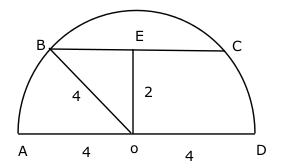Question 27

# On a semicircle with diameter AD, chord BC is parallel to the diameter. Further; AD and BC are separated by 2cm, while AD has length 8. What is the length of BC?

SolutionIn the triangle BEO, BE = $$\sqrt{4^2-2^2}$$ = $$2\sqrt3$$

==> BC = $$4\sqrt3$$